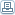# How Accurate Is Your Multimeter And What Is True Rms?

 By: Bob PrevilleEvery electrical technician knows the difference between DC (Direct Current) and AC (Alternating Current). Every electrical technician also realizes the importance of taking accurate current measurements to protect conductors from exceeding their insulators' ability to withstand heat or assuring devices under power work properly. However, does every electrical technician realize that electrical current measurements aren't always what they appear to be?Direct Current (DC) is straightforward. When we use a multimeter to measure direct current, it is what it is. However, the plot thickens when we are dealing with Alternating Current (AC). AC current travels back and forth down a conductor and can best be described in graphical format. The most common graphical description of AC current is a sine wave. Because the amplitude of the sine wave continuously changes over the wave period (one complete cycle), at any given point in time, a current measurement would not be the same. Therefore, how do we accurately measure AC Current?One method to measure AC current would be take current measurements at increments across one complete cycle and average them together. This would give us an average value of the current. If the current is a perfect sine wave, mathematically, the average value is always 0.636 times the value of the peak amplitude.Another method to measure current is based on the current's ability to perform work when applied to a resistive load. The laws of physics tell us that when current passes through a resistive load, it dissipates energy in the form of heat, mechanical motion, radiation or other forms of energy. If the resistive load is a heating element and the resistive load stays constant, then the laws of physics tell us that the heat produced is directly proportionate to the current passing through the load. Therefore, if we measure the heat, we will know the current.Mathematically, the relationship between heat and current is such that the heat produced is proportional to the square of the current applied to a resistance.(Power or Heat) = (Current) ^2 * (Resistance)If the current is continuously changing, as in AC current, the heat produced is proportional to the average (or mean) of the square of the current applied to a resistance:(Power or Heat) = Average [ (Current) ^2 * (Resistance) ]Using algebra, the above formula can be rewritten to read:Current = Square Root [ (Power or Heat) / (Resistance) ]AND this is called the Root Mean Square Current or RMS Current.For AC currents that are graphically represented by a sine wave, the RMS current will always be 0.707 times the peak current. With that said, we can calculate current by multiplying peak measurements by 0.707 if the current is a perfect sine wave. However, perfect sine waves are rare in most commercial and industrial applications. This is because resistive loads in commercial applications are not linear which results in unpredictable or variable current requirements.In order to get a True RMS measurement, we can measure the heat dissipated by a constant resistive load and perform the above calculations. The result is a True RMS measurement.Now that we got all the technical discussion out of the way, which is the best method to calculate current? Should we 1.) measure a current average 2.) multiply current peaks by 0.707 to get an RMS current, or 3.) measure the heat from a resistor and calculate a True RMS current value?Although Global Test Supply sells multimeters that can calculate current using any of the above methods, the most accurate way to calculate current in my opinion is a True RMS method. Average current values often are 40% less than True RMS values and that could mean the difference between blown circuit breakers, malfunctioning motors, or worst case, potential fire hazards. True RMS multimeters only cost about 20-30% more than the alternative. How much is an accurate current reading worth to you?